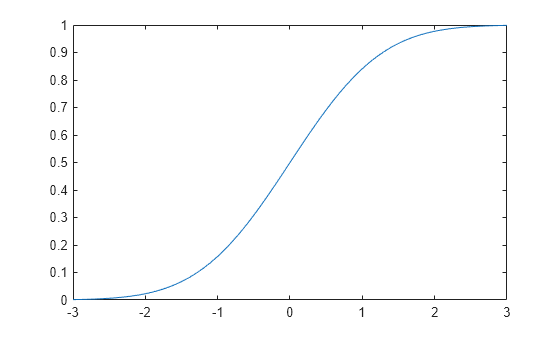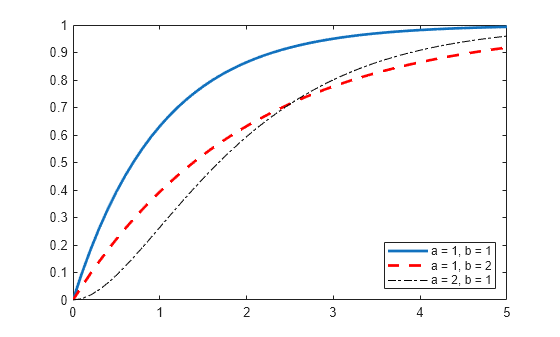# cdf

## 语法

``y = cdf('name',x,A)``
``y = cdf('name',x,A,B)``
``y = cdf('name',x,A,B,C)``
``y = cdf('name',x,A,B,C,D)``
``y = cdf(pd,x)``
``y = cdf(___,'upper')``

## 说明

``y = cdf('name',x,A)` 基于 `x` 中的值计算并返回由 `'name'` 和分布参数 `A` 指定的单参数分布族的累积分布函数 (cdf) 值。`

``y = cdf('name',x,A,B)` 基于 `x` 中的值计算并返回由 `'name'` 以及分布参数 `A` 和 `B` 指定的双参数分布族的 cdf。`
``y = cdf('name',x,A,B,C)` 基于 `x` 中的值计算并返回由 `'name'` 以及分布参数 `A`、`B` 和 `C` 指定的三参数分布族的 cdf。`
``y = cdf('name',x,A,B,C,D)` 基于 `x` 中的值计算并返回由 `'name'` 以及分布参数 `A`、`B`、`C` 和 `D` 指定的四参数分布族的 cdf。`

``y = cdf(pd,x)` 基于 `x` 中的值计算并返回概率分布对象 `pd` 的 cdf。`
``y = cdf(___,'upper')` 使用可更精确计算极值上尾概率的算法返回 cdf 的补函数。`'upper'` 可以跟在上述语法中的任何输入参数之后。`

## 示例

```mu = 0; sigma = 1; pd = makedist('Normal','mu',mu,'sigma',sigma);```

`x = [-2,-1,0,1,2];`

`y = cdf(pd,x)`
```y = 1×5 0.0228 0.1587 0.5000 0.8413 0.9772 ```

y 中的每个值对应于输入向量 x 中的一个值。例如，在值 x 等于 1 时，对应的 cdf 值 y 等于 0.8413。

`y2 = cdf('Normal',x,mu,sigma)`
```y2 = 1×5 0.0228 0.1587 0.5000 0.8413 0.9772 ```

cdf 值与使用概率分布对象计算的值相同。

```lambda = 2; pd = makedist('Poisson','lambda',lambda);```

`x = [0,1,2,3,4];`

`y = cdf(pd,x)`
```y = 1×5 0.1353 0.4060 0.6767 0.8571 0.9473 ```

y 中的每个值对应于输入向量 x 中的一个值。例如，在值 x 等于 3 时，对应的 cdf 值 y 等于 0.8571。

`y2 = cdf('Poisson',x,lambda)`
```y2 = 1×5 0.1353 0.4060 0.6767 0.8571 0.9473 ```

cdf 值与使用概率分布对象计算的值相同。

`pd = makedist('Normal')`
```pd = NormalDistribution Normal distribution mu = 0 sigma = 1 ```

```x = -3:.1:3; p = cdf(pd,x);```

`plot(x,p)``pd_gamma = makedist('Gamma')`
```pd_gamma = GammaDistribution Gamma distribution a = 1 b = 1 ```
`pd_12 = makedist('Gamma','a',1,'b',2)`
```pd_12 = GammaDistribution Gamma distribution a = 1 b = 2 ```
`pd_21 = makedist('Gamma','a',2,'b',1)`
```pd_21 = GammaDistribution Gamma distribution a = 2 b = 1 ```

```x = 0:.1:5; cdf_gamma = cdf(pd_gamma,x); cdf_12 = cdf(pd_12,x); cdf_21 = cdf(pd_21,x);```

```figure; J = plot(x,cdf_gamma); hold on; K = plot(x,cdf_12,'r--'); L = plot(x,cdf_21,'k-.'); set(J,'LineWidth',2); set(K,'LineWidth',2); legend([J K L],'a = 1, b = 1','a = 1, b = 2','a = 2, b = 1','Location','southeast'); hold off;``````t = trnd(3,100,1); obj = paretotails(t,0.1,0.9); [p,q] = boundary(obj)```
```p = 2×1 0.1000 0.9000 ```
```q = 2×1 -1.8487 2.0766 ```

`cdf(obj,q)`
```ans = 2×1 0.1000 0.9000 ```

## 输入参数

`'name'`分布输入参数 `A`输入参数 `B`输入参数 `C`输入参数 `D`
`'Beta'`Beta Distributiona 第一个形状参数b 第二个形状参数
`'Binomial'`Binomial Distributionn 试验次数p 每次试验成功的概率
`'BirnbaumSaunders'`Birnbaum-Saunders Distributionβ 尺度参数γ 形状参数
`'Burr'`Burr Type XII Distributionα 尺度参数c 第一个形状参数k 第二个形状参数
`'Chisquare'`Chi-Square Distributionν 自由度
`'Exponential'`Exponential Distributionμ 均值
`'Extreme Value'`Extreme Value Distributionμ 位置参数σ 尺度参数
`'F'`F Distributionν1 分子自由度ν2 分母自由度
`'Gamma'`Gamma Distributiona 形状参数b 尺度参数
`'Generalized Extreme Value'`Generalized Extreme Value Distributionk 形状参数σ 尺度参数μ 位置参数
`'Generalized Pareto'`Generalized Pareto Distributionk 尾部指数（形状）参数σ 尺度参数μ 阈值（位置）参数
`'Geometric'`Geometric Distributionp 概率参数
`'HalfNormal'`Half-Normal Distributionμ 位置参数σ 尺度参数
`'Hypergeometric'`Hypergeometric Distributionm 总体的大小k 总体中具有所需特征的项数n 抽取的样本数量
`'InverseGaussian'`Inverse Gaussian Distributionμ 尺度参数λ 形状参数
`'Logistic'`Logistic Distributionμ 均值σ 尺度参数
`'LogLogistic'`Loglogistic Distributionμ 对数值的均值σ 对数值的尺度参数
`'Lognormal'`Lognormal Distributionμ 对数值的均值σ 对数值的标准差
`'Nakagami'`Nakagami Distributionμ 形状参数ω 尺度参数
`'Negative Binomial'`Negative Binomial Distributionr 成功次数p 单个试验的成功概率
`'Noncentral F'`Noncentral F Distributionν1 分子自由度ν2 分母自由度δ 非中心参数
`'Noncentral t'`Noncentral t Distributionν 自由度δ 非中心参数
`'Noncentral Chi-square'`Noncentral Chi-Square Distributionν 自由度δ 非中心参数
`'Normal'`正态分布μ 均值 σ 标准差
`'Poisson'`泊松分布λ 均值
`'Rayleigh'`Rayleigh Distributionb 尺度参数
`'Rician'`Rician Distributions 非中心参数σ 尺度参数
`'Stable'`Stable Distributionα 第一个形状参数β 第二个形状参数γ 尺度参数δ 位置参数
`'T'`Student's t Distributionν 自由度
`'tLocationScale'`t Location-Scale Distributionμ 位置参数σ 尺度参数ν 形状参数
`'Uniform'`Uniform Distribution (Continuous)a 下部端点（最小值）b 上部端点（最大值）
`'Discrete Uniform'`Uniform Distribution (Discrete)n 最大可观测值
`'Weibull'`Weibull Distributiona 尺度参数b 形状参数

`makedist`使用指定的参数值创建一个概率分布对象。
`fitdist`对样本数据进行概率分布对象拟合。

`paretotails`创建一个分段分布对象，它在尾部具有广义帕累托分布。

## 输出参数

cdf 值，以标量值或标量值组成的数组形式返回。在经过任何必要的标量扩展后，`y` 的大小与 `x` 相同。`y` 中的每个元素均为由分布参数（`A``B``C``D`）中的对应元素或概率分布对象 (`pd`) 指定的分布的 cdf 值，其值在 `x` 中的对应元素处进行计算。